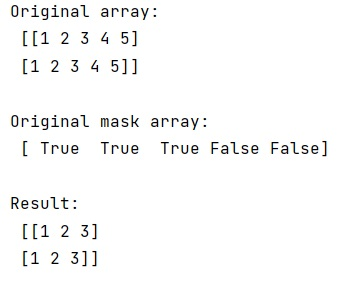# Select elements of numpy array via boolean mask array

Learn, how to select elements of numpy array via boolean mask array in Python?
Submitted by Pranit Sharma, on February 16, 2023

NumPy is an abbreviated form of Numerical Python. It is used for different types of scientific operations in python. Numpy is a vast library in python which is used for almost every kind of scientific or mathematical operation. It is itself an array which is a collection of various methods and functions for processing the arrays.

## NumPy Array - Select elements via boolean mask array

Suppose we are given a 2D numpy array with n columns and a boolean mask array of length n. We need to compare both arrays and create another array such that the values of the 2D array corresponding to the True values in the boolean mask array will be set as the elements of the new array.

For this purpose, we need to use the indexing technique. Note that there must be a ndarray to apply this type of indexing.

Let us understand with the help of an example,

## Python code to select elements of numpy array via boolean mask array

```# Import numpy
import numpy as np

# Creating a numpy array
arr = np.array([[1,2,3,4,5], [1,2,3,4,5]])

# Display original array
print("Original array:\n",arr,"\n")

# Creating a boolean mask array
boo = np.array([True, True, True, False, False])

# Creating a new array based on True values and arr
res = arr[:,boo]

# Display Result
print("Result:\n",res)
```

Output: